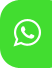## Product Detail## Ignou Solved Assignment for MECE-001: Econometric methods

University  IGNOU
Service Type Solved Assignment
Course MA(Economics)
Semester
Short Name or Subject Code MECE-001: ECONOMETRIC METHODS
Product MA(Economics) of Solved Assignment (IGNOU)
Pattern
Price
Click to view price

Section A

1. In the case of a two-variable regression model show that TSS = ESS + RSS. Use appropriate diagram to explain your result. In this context define the concept of R-squared and interpret it.

2.  a) What is meant by identification problem in a simultaneous equation model?

b) In the following two-equation system check the identification status of both the equations.

𝑌1 =∝1+∝2 𝑌2 + 𝛽1𝑍2 + 𝑢1 𝑌2 = 𝛽2 + 𝛽3𝑌1 + 𝛽4𝑍1 + 𝛽5𝑍2 + 𝑢2 c)

Explain how the first equation in the above model can be estimated.

Section B

3. What are the limitations of the linear probability model? Explain how the logit model can be used to overcome these limitations.

4. What are its consequences of heteroscedasticity? How do you detect it? Suggest a method you would follow to remove the heteroscedasticity problem in a dataset.

5. What are the advantages of dummy variables in a regression model? What is dummyvariable trap? Formulate a regression model with intercept and slope dummies.

6. Suppose the explanatory variable in a regression model is measured with error. What are its consequences? What steps will you take to solve the problem?

7. Write short notes on the following:

a) Sampling distribution and standard error

b) General Least Squares model